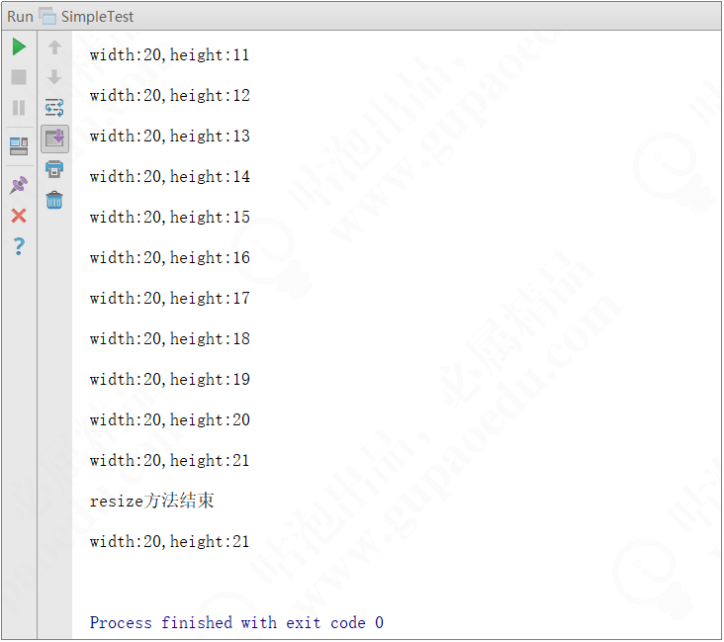# 软件架构设计原则之里氏替换原则Tom弹架构

（1）子类可以实现父类的抽象方法，但不能覆盖父类的非抽象方法。

（2）子类可以增加自己特有的方法。

（3）当子类的方法重载父类的方法时，方法的前置条件（即方法的输入/入参）要比父类方法的输入参数更宽松。

（4）当子类的方法实现父类的方法时（重写/重载或实现抽象方法），方法的后置条件（即方法的输出/返回值）要比父类更严格或与父类一样。

public class JavaDiscountCourse extends JavaCourse {    public JavaDiscountCourse(Integer id, String name, Double price) {        super(id, name, price);    }    public Double getDiscountPrice(){        return super.getPrice() * 0.61;    }}

（1）约束继承泛滥，是开闭原则的一种体现。

（2）加强程序的健壮性，同时变更时也可以做到非常好的兼容性，提高程序的可维护性和扩展性，降低需求变更时引入的风险。

public class Rectangle {    private long height;    private long width;    @Override    public long getWidth() {        return width;    }    @Override    public long getLength() {        return length;    }    public void setLength(long length) {        this.length = length;    }    public void setWidth(long width) {        this.width = width;    }}

public class Square extends Rectangle {    private long length;    public long getLength() {        return length;    }    public void setLength(long length) {        this.length = length;    }    @Override    public long getWidth() {        return getLength();    }    @Override    public long getHeight() {        return getLength();    }    @Override    public void setHeight(long height) {        setLength(height);    }    @Override    public void setWidth(long width) {        setLength(width);    }}

public static void resize(Rectangle rectangle){    while (rectangle.getWidth() >= rectangle.getHeight()){        rectangle.setHeight(rectangle.getHeight() + 1);        System.out.println("width:"+rectangle.getWidth() + ",height:"+rectangle.getHeight());    }    System.out.println("resize方法结束" +            "\nwidth:"+rectangle.getWidth() + ",height:"+rectangle.getHeight());}

public static void main(String[] args) {    Rectangle rectangle = new Rectangle();    rectangle.setWidth(20);    rectangle.setHeight(10);    resize(rectangle);}public static void main(String[] args) {    Square square = new Square();    square.setLength(10);    resize(square);}

public interface Quadrangle {    long getWidth();    long getHeight();}

public class Rectangle implements Quadrangle {    private long height;    private long width;    @Override    public long getWidth() {        return width;    }    public long getHeight() {        return height;    }    public void setHeight(long height) {        this.height = height;    }    public void setWidth(long width) {        this.width = width;    }}

public class Square implements Quadrangle {    private long length;    public long getLength() {        return length;    }    public void setLength(long length) {        this.length = length;    }    @Override    public long getWidth() {        return length;    }    @Override    public long getHeight() {        return length;    }}## 评论